## Machine Learning & Data Science Algorithmic Programming Section

Algorithmic Quantitative Python - R programming for Data Science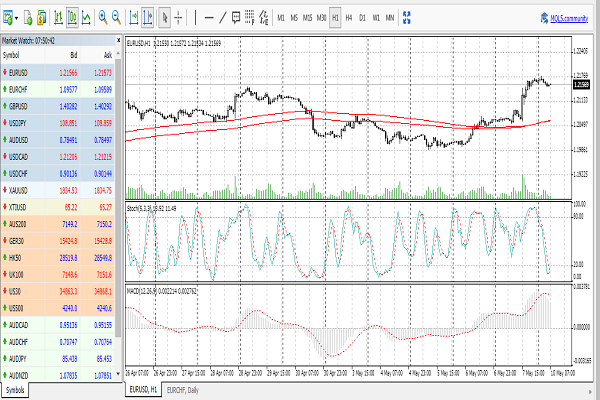## Python & R programming

Quant Algorithmic Programming

Using hypothesis generation engineering, naïve bayes, random forest models and multiple machine learning algorithms used for prediction.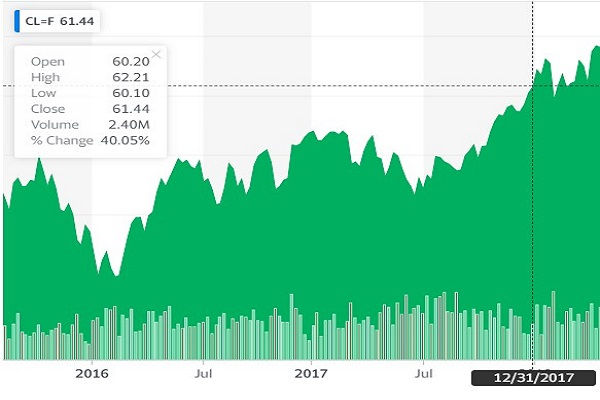## Python programming

Python Algorithmic programming

Python using Numpy, Pandas, Matplotlib, scikit-learn, Keras, Tensorflow, all of this used for Algorithmic Quantitative programming.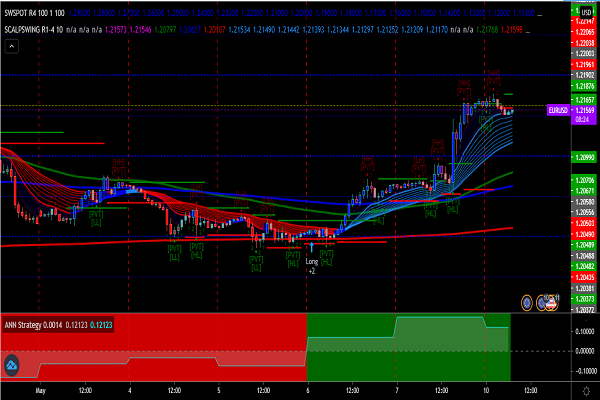## Python programming

Python Algorithmic programming

Supervised/Unsupervised learning, Classification, Clustering, Association Detection, Anomaly Detection, Dimensionality Reduction, Regression.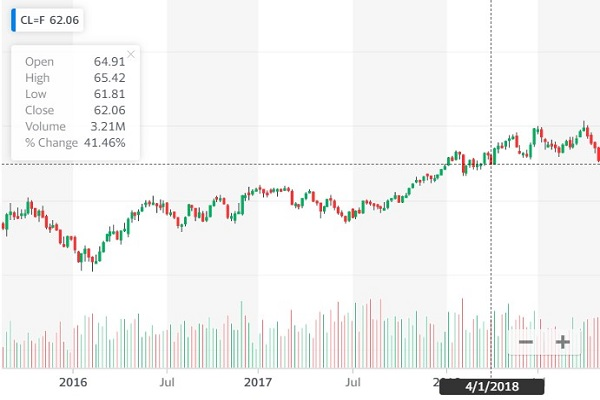## Python & R programming

Quanta Algorithmic Programming

Naive Bayes, K-nearest neighbours, Support Vector Machines, Artificial Neural Networks, K-means, Hierarchical clustering, Principal Components Analysis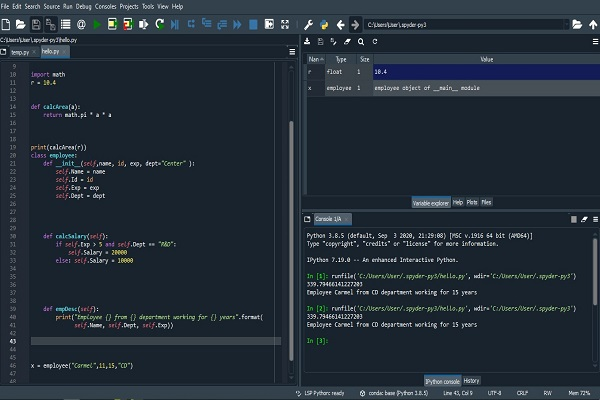## Python programming

Python Algorithmic programming

Python using Linear regression, Logistics regression, Random variables, Bayes theorem, Bias-variance tradeoff .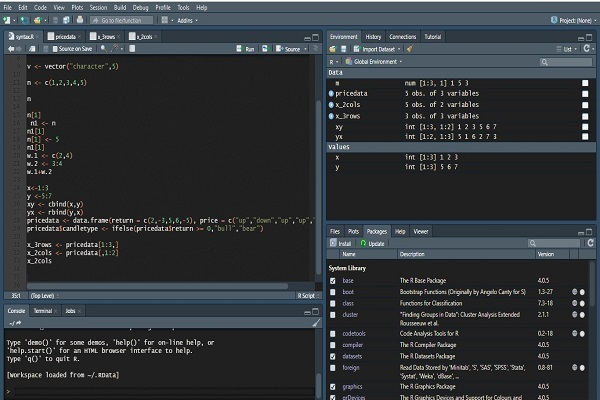## Python programming

Python programming

Python using Alpha and Beta coefficients, regression’s explanatory power R^2, Markowitz Efficient frontier, Sharpe ratio, Monte Carlo simulations .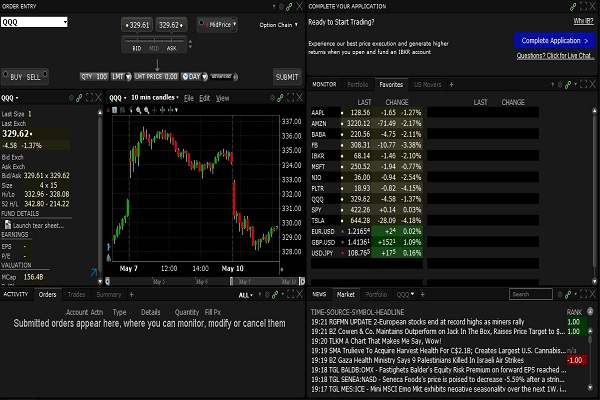## R programming

R Algorithmic Programming

Using Data Preprocessing, Linear Regression, Logistic Regression, Support Vector Machines, K-Means Clustering, Ensemble Learning, Natural Language Processing, Neural Nets.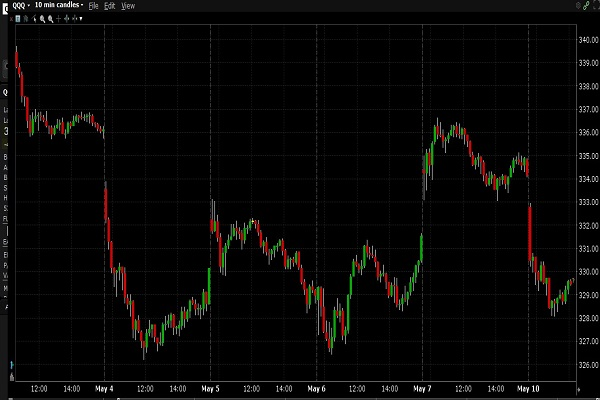## Python programming

Python Algorithmic programming

Python using pandas-datareader and Quandl, Pandas Time Series Analysis Techniques, Volatility and Securities Risk, Efficient Frontier and Markowitz Optimization.## Python programming

Python Algorithmic programming

Python using EWMA (Exponentially Weighted Moving Average), Statsmodels, ETS (Error-Trend-Seasonality), ARIMA (Auto-regressive Integrated Moving Averages), Auto Correlation Plots and Partial Auto Correlation Plots, Sharpe Ratio .